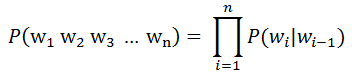Advanced Database Management System - Tutorials and Notes: Natural Language Processing MCQ based Quiz

## Natural Language Processing MCQ with answers

1. Given a sentence S="w1 w2 w3 … wn", to compute the likelihood of S using a bigram model. How would you compute the likelihood of S?
a. Calculate the conditional probability of each word in the sentence given the preceding word and add the resulting numbers
b. Calculate the conditional probability of each word in the sentence given the preceding word and multiply the resulting numbers
c. Calculate the conditional probability of each word given all preceding words in a sentence and add the resulting numbers
d. Calculate the conditional probability of each word given all preceding words in a sentence and multiply the resulting numbers
 Answer: (b) As per Bi-gram model, probability of certain sequence using the Markov assumption is expressed as follows;The Right Hand Side of the equation can be applied for a sentence “ I am Sam ” as follows; P(“ I am sam ”) = P(I|) * P(am|I) * P(Sam|am) * P(|Sam)

2. In the sentence, “They bought a blue house”, the underlined part is an example of _____.
a. Noun phrase
b. Verb phrase
c. Prepositional phrase
 Answer: (a) A noun phrase is a word or group of words containing a noun and functioning in a sentence as subject, object, or prepositional object. It is a phrase that has a noun as its head or performs the same grammatical function as a noun.

3. Consider the following Context-Free Grammar G;
 S → A B               (1) S → A S C           (2) B → A C D          (3) A → a                  (4) C → c                   (5) D → d                  (6)
Which of the following strings belong to the language defined by the above grammar?
b. aacd
c. aaacdc
d. aaaaacdcc
 Answer: (b) and (c) aacd can be derived through the following derivation; S ⇒ AB ⇒ aB ⇒ aACD ⇒ aaCD ⇒ aacD ⇒ aacd [rule 1 ⇒ rule 4 ⇒ rule 3 ⇒ rule 4 ⇒ rule 5 ⇒ rule 6] For aaacdc, please try.

4. Let us assume that CorpA is a corpus of English with approximately 560 million tokens. Following are the counts of unigrams and bigrams from the corpus;
 snow purple purple snow 30250 12321 0
Find the probability of P(snow|purple) using maximum likelihood estimation without smoothing.
a. 12321
b. 30250
c. 0.4073
d. 0
 Answer: (d) Using Maximum Likelihood Estimate, P(snow|purple) = count(purple snow) / count(purple) = 0/12321 = 0.

5. 4-grams are better than trigrams for part-of-speech tagging.
a. TRUE
b. FALSE
 Answer: (b) FALSE. There is not generally enough data for 4-grams to outperform trigrams. n-grams bigger than tri-grams is not a solution as the n-grams would often not occur in the training data, making the associated probabilities hard to estimate.

**********